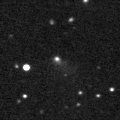# \$B%j%K%"WB@1(B

236P/LINEAR (2010)###\$B%W%m%U%#!<%k(B

 \$BId9f(B 236P/2010 K1 \$B8!=PF|(B 2010\$BG/(B5\$B7n(B20\$BF|(B \$B8!=P8wEY(B 21.5\$BEy(B \$B8!=P J. V. Scotti (Spacewatch)

###\$B###\$B50F;MWAG(B

```                    Epoch = 2010 Sept. 1.0 TT
T = 2010 Sept. 8.8213 TT         Peri. = 119.3369
e = 0.509100                     Node  = 245.6704  2000.0
q = 1.831352 AU                  Incl. =  16.3321
a =  3.730598 AU    n = 0.1367843    P =   7.21 years
```

###\$B@1?^(B###\$B8wEYJQ2=(B

```        m1 = 15.0 + 5 log\$B&\$(B + 10.0 log r
```##### \$B50F;MWAG\$O!"(BIAUC 9149\$B\$K7G:\\$5\$l\$?\$b\$N\$G\$9!#(B \$B@1?^\$O%9%F%i%J%S%2!<%?(B Ver.8 (\$B%"%9%H%m%"!<%D(B) \$B\$G:n@.\$7\$?\$b\$N\$G\$9!#(B \$B8wEY%0%i%U\$O(BComet for Windows\$B\$G:n@.\$7\$?\$b\$N\$G\$9!#(B